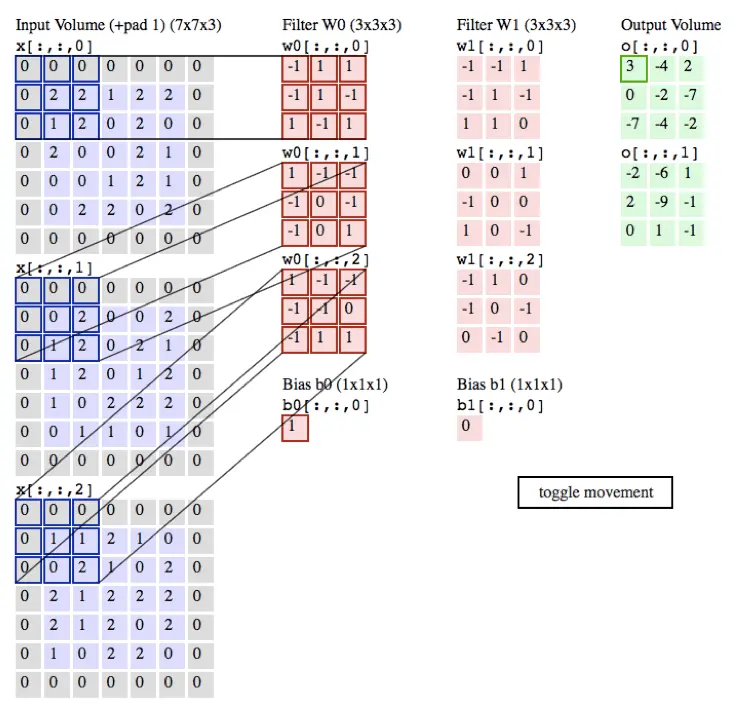## PyTorch-专知-链路化知识-2、< 快速理解系列 (一): 图文 代码, 让你快速理解 CNN >

### 2、< 快速理解系列(一): 图文+代码, 让你快速理解CNN >

#### 一、神经网络（Neural Network）

• 要弄清楚什么是卷积神经网络，首先让我们了解一下什么是神经网络。在上一篇文章的内容中，我们实现了基本神经网络的构建和训练（具体实现细节参考上一篇文章的代码）。神经网络的输入是一个向量，然后在一系列的隐层中对它做变换。每个隐层都是由若干的神经元组成，每个神经元都与前一层中的某些神经元连接。同一隐层中，神经元相互独立不进行任何连接。某些层会将神经元全部连接,称为全连接层 ***
• 神经元

下图是一个激活函数为sigmoid函数的神经元• 神经网络其实就是按照一定规则连接起来的多个神经元。下图展示了两个全连接(full connected, FC)神经网络。#### 二、卷积神经网络（Convolutional Neural Network）

• 卷积神经网络（Convolutional Neural Network）简称CNN。它是近些年逐步兴起的一种人工神经网络结构, 因为利用卷积神经网络在图像和语音识别方面能够给出更优预测结果, 这一种技术也被广泛的传播和应用.
• 在图像处理中，往往把图像表示为像素的向量，比如一个1000×1000的图像，可以表示为一个1000000的向量。在以上提到的神经网络中，如果隐含层数目与输入层一样，即也是1000000时，那么输入层到隐含层的参数数据为1000000×1000000=10^12，这样就太多了，基本没法训练。所以图像处理要想练成神经网络大法，必先减少参数加快速度。 #### 1、局部感知
• 卷积神经网络有两种方式可以降低参数数目，第一种方法叫做局部感知野（receptive field）。一般认为人对外界的认知是从局部到全局的，对于一张图像来说, 同样是局部的像素联系较为紧密，而距离较远的像素相关性较弱。因而，每个神经元其实没有必要对全局图像进行感知，只需要对局部进行感知，然后在更高层将局部的信息综合起来就得到全局的信息即可。网络部分连通的思想，也是受启发于生物学里面的视觉系统结构。视觉皮层的神经元就是局部接受信息的（即这些神经元只响应某些特定区域的刺激）。

• 感受野（receptive field），它的尺寸是一个超参数（其实就是滤波器的空间尺）。在深度方向上，这个连接的大小总是和输入量的深度相等。需要再次强调的是，我们对待空间维度（宽和高）与深度维度是不同的：连接在空间（宽高）上是局部的，但是在深度上总是和输入数据的深度一致。#### 2、参数共享

• 首先，输出数据的深度是一个超参数：它和使用的滤波器的数量一致，而每个滤波器在输入数据中寻找一些不同的东西。举例来说，如果第一个卷积层的输入是原始图像，那么在深度维度上的不同神经元将可能被不同方向的边界，或者是颜色斑点激活。我们将这些沿着深度方向排列、感受野相同的神经元集合称为深度列（depth column），也有人使用纤维（fibre）来称呼它们。
• 其次，在滑动滤波器的时候，必须指定步长。当步长为1，滤波器每次移动1个像素。当步长为2（或者不常用的3，或者更多，这些在实际中很少使用），滤波器滑动时每次移动2个像素。这个操作会让输出数据体在空间上变小。
• 在下文可以看到，有时候将输入数据用0在边缘处进行填充是很方便的。这个零填充（zero-padding）的尺寸是一个超参数。零填充有一个良好性质，即可以控制输出数据体的空间尺寸（最常用的是用来保持输入数据体在空间上的尺寸，这样输入和输出的宽高都相等）。#### 3、Pooling#### 4、卷积神经网络的构建

• 层的排列规矩

• 卷积神经网络最常见的形式就是将一些卷积层和ReLU层放在一起，其后紧跟池化层，然后重复如此直到图像在空间上被缩小到一个足够小的尺寸，在某个地方过渡成成全连接层也较为常见。最后的全连接层得到输出，比如分类评分等。换句话说，最常见的卷积神经网络结构如下：

INPUT -> [[CONV -> RELU] * N -> POOL?] * M -> [FC -> RELU] * K -> FC 其中*指的是重复次数，POOL?指的是一个可选的汇聚层。其中N >=0,通常N<=3,M>=0,K>=0,通常K<3。

#### 三、卷积神经网络CNN的PyTorch实现

• 使用Mnist数据集实现的CNN

• 导入包

import torch
import torch.nn as nn
import torchvision.datasets as normal_datasets
import torchvision.transforms as transforms
from torch.autograd import Variable

• 下载数据集


num_epochs = 1
batch_size = 100
learning_rate = 0.001

#将数据处理成Variable, 如果有GPU, 可以转成cuda形式
def get_variable(x):
x = Variable(x)
return x.cuda() if torch.cuda.is_available() else x

#从torchvision.datasets中加载一些常用数据集
train_dataset = normal_datasets.MNIST(
root='./mnist/',                 # 数据集保存路径
train=True,                      # 是否作为训练集
transform=transforms.ToTensor(), # 数据如何处理, 可以自己自定义
download=True)                   # 路径下没有的话, 可以下载

# 见数据加载器和batch
test_dataset = normal_datasets.MNIST(root='./mnist/',
train=False,
transform=transforms.ToTensor())


• 处理数据，使用 DataLoader 进行batch训练

train_loader = torch.utils.data.DataLoader(dataset=train_dataset,
batch_size=batch_size,
shuffle=True)

test_loader = torch.utils.data.DataLoader(dataset=test_dataset,
batch_size=batch_size,
shuffle=False)


• 建立计算图模型
# 两层卷积
class CNN(nn.Module):
def __init__(self):
super(CNN, self).__init__()
# 使用序列工具快速构建
self.conv1 = nn.Sequential(
nn.Conv2d(1, 16, kernel_size=5, padding=2),
nn.BatchNorm2d(16),
nn.ReLU(),
nn.MaxPool2d(2))
self.conv2 = nn.Sequential(
nn.Conv2d(16, 32, kernel_size=5, padding=2),
nn.BatchNorm2d(32),
nn.ReLU(),
nn.MaxPool2d(2))
self.fc = nn.Linear(7 * 7 * 32, 10)

def forward(self, x):
out = self.conv1(x)
out = self.conv2(out)
out = out.view(out.size(0), -1)  # reshape
out = self.fc(out)
return out

cnn = CNN()
if torch.cuda.is_available():
cnn = cnn.cuda()

• 定义优化器optimizer和损失  # 选择损失函数和优化方法 loss_func = nn.CrossEntropyLoss() optimizer = torch.optim.Adam(cnn.parameters(), lr=learning_rate) 
• 进行batch训练

for epoch in range(num_epochs):
for i, (images, labels) in enumerate(train_loader):
images = get_variable(images)
labels = get_variable(labels)

outputs = cnn(images)
loss = loss_func(outputs, labels)
optimizer.zero_grad()
loss.backward()
optimizer.step()

if (i + 1) % 100 == 0:
print('Epoch [%d/%d], Iter [%d/%d] Loss: %.4f'
% (epoch + 1, num_epochs, i + 1, len(train_dataset) // batch_size, loss.data))
# 测试模型
cnn.eval()  # 改成测试形态, 应用场景如: dropout
correct = 0
total = 0
for images, labels in test_loader:
images = get_variable(images)
labels = get_variable(labels)
outputs = cnn(images)
_, predicted = torch.max(outputs.data, 1)
total += labels.size(0)
correct += (predicted == labels.data).sum()

print(' 测试 准确率: %d %%' % (100 * correct / total))

# Save the Trained Model
torch.save(cnn.state_dict(), 'cnn.pkl')



#### 四、CNN常见模型之ResNet

ResNet——MSRA何凯明团队的Residual Networks，在2015年ImageNet上大放异彩，在ImageNetclassificationdetectionlocalization以及COCOdetectionsegmentation上均斩获了第一名的成绩，而且Deep Residual Learning for Image Recognition也获得了CVPR2016best paper，实在是实至名归。论文地址

import torch
import torch.nn as nn
import torchvision.datasets as normal_datasets
import torchvision.transforms as transforms
from torch.autograd import Variable

num_epochs = 1
lr = 0.001

def get_variable(x):
x = Variable(x)
return x.cuda() if torch.cuda.is_available() else x

# 图像预处理
transform = transforms.Compose([
transforms.Scale(40),
transforms.RandomHorizontalFlip(),
transforms.RandomCrop(32),
transforms.ToTensor()])

# 加载CIFAR-10
train_dataset = normal_datasets.CIFAR10(root='./cifar_10/',
train=True,
transform=transform,
download=True)

test_dataset = normal_datasets.CIFAR10(root='./cifar_10/',
train=False,
transform=transforms.ToTensor())

train_loader = torch.utils.data.DataLoader(dataset=train_dataset,
batch_size=100,
shuffle=True)

test_loader = torch.utils.data.DataLoader(dataset=test_dataset,
batch_size=100,
shuffle=False)

# 3x3 卷积
def conv3x3(in_channels, out_channels, stride=1):
return nn.Conv2d(in_channels, out_channels, kernel_size=3,
stride=stride, padding=1, bias=False)

# Residual Block
class ResidualBlock(nn.Module):
def __init__(self, in_channels, out_channels, stride=1, downsample=None):
super(ResidualBlock, self).__init__()
self.conv1 = conv3x3(in_channels, out_channels, stride)
self.bn1 = nn.BatchNorm2d(out_channels)
self.relu = nn.ReLU(inplace=True)
self.conv2 = conv3x3(out_channels, out_channels)
self.bn2 = nn.BatchNorm2d(out_channels)
self.downsample = downsample

def forward(self, x):
residual = x
out = self.conv1(x)
out = self.bn1(out)
out = self.relu(out)
out = self.conv2(out)
out = self.bn2(out)
if self.downsample:
residual = self.downsample(x)
out += residual
out = self.relu(out)
return out

# ResNet Module
class ResNet(nn.Module):
def __init__(self, block, layers, num_classes=10):
super(ResNet, self).__init__()
self.in_channels = 16
self.conv = conv3x3(3, 16)
self.bn = nn.BatchNorm2d(16)
self.relu = nn.ReLU(inplace=True)
self.layer1 = self.make_layer(block, 16, layers)
self.layer2 = self.make_layer(block, 32, layers, 2)
self.layer3 = self.make_layer(block, 64, layers, 2)
self.avg_pool = nn.AvgPool2d(8)
self.fc = nn.Linear(64, num_classes)

def make_layer(self, block, out_channels, blocks, stride=1):
downsample = None
if (stride != 1) or (self.in_channels != out_channels):
downsample = nn.Sequential(
conv3x3(self.in_channels, out_channels, stride=stride),
nn.BatchNorm2d(out_channels))
layers = []
layers.append(block(self.in_channels, out_channels, stride, downsample))
self.in_channels = out_channels
for i in range(1, blocks):
layers.append(block(out_channels, out_channels))
return nn.Sequential(*layers)

def forward(self, x):
out = self.conv(x)
out = self.bn(out)
out = self.relu(out)
out = self.layer1(out)
out = self.layer2(out)
out = self.layer3(out)
out = self.avg_pool(out)
out = out.view(out.size(0), -1)
out = self.fc(out)
return out

resnet = ResNet(ResidualBlock, [2, 2, 2, 2])
if torch.cuda.is_available():
resnet = resnet.cuda()

loss_func = nn.CrossEntropyLoss()
optimizer = torch.optim.Adam(resnet.parameters(), lr=lr)

# 训练
for epoch in range(num_epochs):
for i, (images, labels) in enumerate(train_loader):
images = get_variable(images)
labels = get_variable(labels)

outputs = resnet(images)
loss = loss_func(outputs, labels)
optimizer.zero_grad()
loss.backward()
optimizer.step()

if (i + 1) % 100 == 0:
print("Epoch [%d/%d], Iter [%d/%d] Loss: %.4f" % (epoch + 1, num_epochs, i + 1, 500, loss.data))

# 衰减学习率
if (epoch + 1) % 20 == 0:
lr /= 3
optimizer = torch.optim.Adam(resnet.parameters(), lr=lr)

# 测试
correct = 0
total = 0
for images, labels in test_loader:
images = get_variable(images)
labels = get_variable(labels)
outputs = resnet(images)
_, predicted = torch.max(outputs.data, 1)
total += labels.size(0)
correct += (predicted == labels.data).sum()

print(' 测试 准确率: %d %%' % (100 * correct / total))

# 保存模型参数
torch.save(resnet.state_dict(), 'resnet.pkl')


Reference：Top